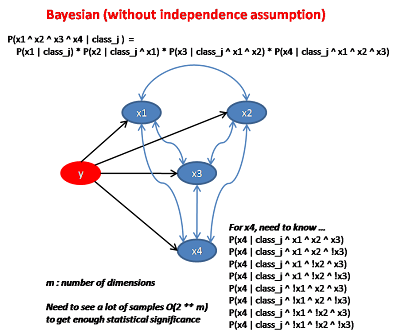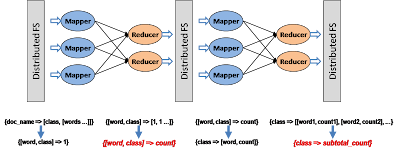## Monday, May 4, 2009

### Machine Learning: Probabilistic Model

Probabilistic model is a very popular approach of “model-based learning” based on Bayesian theory. Under this approach, all input attributes is binary (for now) and the output is categorical.

Here, we are given a set of data with structure [x1, x2 …, y] is presented. (in this case y is the output). The learning algorithm will learn (from the training set) how to predict the output y for future seen data

We assume there exist a hidden probability distribution from the input attributes to the output. The goal is to learn this hidden distribution and apply it to the input attributes of the later encountered query point to pick the class that has the maximum probability.

Making Prediction

Lets say the possible value of output y is {class_1, class_2, class_3}. Given input [x1, x2, x3, x4], we need to compute the probability of each output class_j, and predict the one which has the highest value.
``max {P(class_j | observed_attributes)}``

According to Bayes theorem, this value is equal to …
``max { P(observed_attributes | class_j) * P(class_j) / P(observed_attributes) }``

The dominator is the same for all class_j, so we can ignore it, so we just need to find
``max { P(observed_attributes | class_j) * P(class_j) }``

P(class_j) is easy to find, we just calculate
``P(class_j) = (samples_of_class_j / total samples)``

Now, lets look at the other term, P(observed_attributes | class_j), from Bayesian theory
``P(x1 ^ x2 ^ x3 ^ x4 | class_j) =P(x1 | class_j) *P(x2 | class_j ^ x1) *P(x3 | class_j ^ x1 ^ x2) *P(x4 | class_j ^ x1 ^ x2 ^ x3)``

Learning the probability distribution

In order to provide all the above terms for the prediction, we need to build the probability distribution model by observing the training data set.

Notice that finding the last term is difficult. Assume x1, x2 ... is binary, there can be 2 ** (m – 1) possible situations to watch. It is very likely that we haven’t seen enough situations in the training data, in this case this term for all class_j will be zero. So a common solution is to start with count = 1 for all possible combinations and increase the count when we see an occurrence in the training data.Bayesian Network

Bayesian Network base on the fact we know certain attributes are clearly independent. By applying this domain knowledge, we draw a dependency graph between attributes. The probability of occurrence of a particular node only depends on the occurrence of its parent nodes and nothing else. To be more precise, nodeA and nodeB (which is not related with a parent-child relationship) doesn't need to be completely independent, they just need to be independent given their parents.

In other words, we don't mean P(A|B) = P(A),
we just need P(A | parentsOfA ^ B) = P(A | parentsOfA)
Therefore P(x4 | class_j ^ x1 ^ x2 ^ x3) = P(x4 | class_j ^ parentsOf_x4)

we only need to find 2 ** p situations of the occurrence combination of the parent nodes where p is the number of parent nodes.Naive Bayes

Naive Bayes takes a step even further by assuming every node is completely independentNote that x1, x2, x3, x4 can be categorical as well. For example, if x1 represents zip code, then P('95110' | class_j) is the same as P(x1 = '95110' | class_j).

What if x1 is a continuous variable ? (say height of a person). The challenge is that a continuous variable has infinite possibility such that the chance of seeing one in the training data is almost zero.

One way to deal with continuous variable is to discretetize it. In other words, we can partition x1 into buckets which has an associated range and assign x1 to the corresponding bucket if it falls into that range.

Another way is for each output class_j, we presume a arbitrary distribution function for x1. (lets say we pick the normal distribution function). So the purpose of the training phase is to figure out the parameter of this distribution function (in this case, it is the mean and standard deviation).

In other words, we use the subset of training data whose output class is class_j. Within this subset, we compute the mean and standard deviation of x1 (height of the person). Later on when we want to compute P(x1 = 6.1 feet | class_j), we just apply the distribution function (plug-in the learned mean and standard deviation).

Note that the choice of the form of the distribution function is quite arbitrary here and it may be simply wrong. So it is important to analyze how x1 affects the output class in order to pick the right distribution function.

Spam Filter Application

Lets walk through an application of the Naive Bayes approach. Here we want to classify a particular email to determine whether it is spam or not.

The possible value of output y is {spam, nonspam}

Given an email: "Hi, how are you doing ?", we need to find ...
max of P(spam | mail) and P(nonspam | mail), which is same as ...
max of P(mail | spam) * P(spam) and P(mail | nonspam) * P(nonspam)

Lets focus in P(mail | spam) * P(spam)

We can view mail as an array of words [w1, w2, w3, w4, w5]
``P(mail | spam) =P(w1='hi' | spam) *P(w2='how' | spam ^ w1='hi') *P(w3='are' | spam ^ w1='hi' ^ w2='how') *...``
We make some naive assumptions here
• Chance of occurrence is independent of preceding words. In other words, P(w2='how' | spam ^ w1='hi') is the same as P(w2='how' | spam)
• Chance of occurrence is independent of word position. P(w2='how' | spam) is the same as (number of 'how' in spam mail) / (number of all words in spam mail)
With these assumptions ...
``P(mail | spam) =(hi_count_in_spam / total_words_in_spam) *(how_count_in_spam / total_words_in_spam) *(are_count_in_spam / total_words_in_spam) *...``
What if we haven't seen the word "how" from the training data ? Then the probability will becomes zero. Here we adjust terms such that a reasonable probability is assigned to unseen words.
``P(mail | spam) =((hi_count_in_spam + 1) / (total_words_in_spam + total_vocabulary)) *((how_count_in_spam + 1) / (total_words_in_spam + total_vocabulary)) *((are_count_in_spam + 1) / (total_words_in_spam + total_vocabulary)) *...``

What we need is the word_count per word/class combination as well as the word_count per class. This can be done through feeding a large number of training sample mails labeled with "spam" or "nonspam" into a learning process.

The learning process can also be done in parallel using a 2-rounds of Map/Reduce.Alternatively, we can also update the counts incrementally as new mail arrives.

#### 1 comment:Nedu said...

Hi Ricky Ho,

Can it be done using couch db view engine? Could you please give a small explanation about the way it could be done in couchdb map/reduce